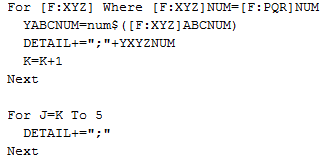# Write line level field values in a single line through a table with the values in fixed number of fields during file creation

By | January 30, 2019

Sometimes while creating/writing to a file we might need to write values from a field in a grid but through a table and not by looping through the grid and the values would be written sequentially in a fixed number of fields in a single line in the file and assigning blank values to the fields in the file if the number of lines in the grid is less than the number of fields in the file. For example, if the number of fields in the file is 5 and the number of lines in the grid is 3, then the 4th and the 5th field in the file would be blank. So refer the below code snippet-Where,

[F:XYZ] is the required table.

ABCNUM is the required field.

“;” is the separator.

Hope this blog helps!